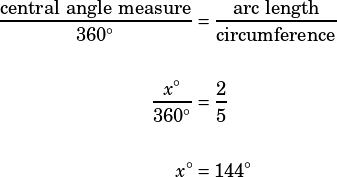Test 8 Section 3 #20

This question is asking you to figure out the measure of a circle’s central angle from information about an arc length. This is a piece of cake if you remember that arc length, sector area (a sector is like a pizza slice shape), and central angle are all tied together via proportion:Lots of circle questions (see some examples in the style of the old SAT here) will simply ask you to solve variations on that proportion.

In this case, when the question tells you that the length of arc BC isof the circle’s circumference, it’s giving you the middle fraction! So we can solve for the value of x easily: